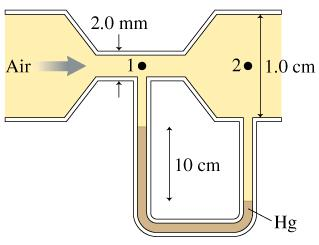# Problem: Air flows through the tube shown in the figure. Assume that air is an ideal fluid. ﻿v1 = 149 m/s.What is the volume flow rate?

###### FREE Expert Solution

Continuity equation:

$\overline{){\mathbf{Q}}{\mathbf{=}}{{\mathbf{A}}}_{{\mathbf{1}}}{{\mathbf{v}}}_{{\mathbf{1}}}{\mathbf{=}}{{\mathbf{A}}}_{{\mathbf{2}}}{{\mathbf{v}}}_{{\mathbf{2}}}{\mathbf{=}}{{\mathbf{A}}}_{{\mathbf{n}}}{{\mathbf{v}}}_{{\mathbf{n}}}}$

Q = v1A1

90% (485 ratings)###### Problem Details

Air flows through the tube shown in the figure. Assume that air is an ideal fluid. ﻿v1 = 149 m/s.What is the volume flow rate?